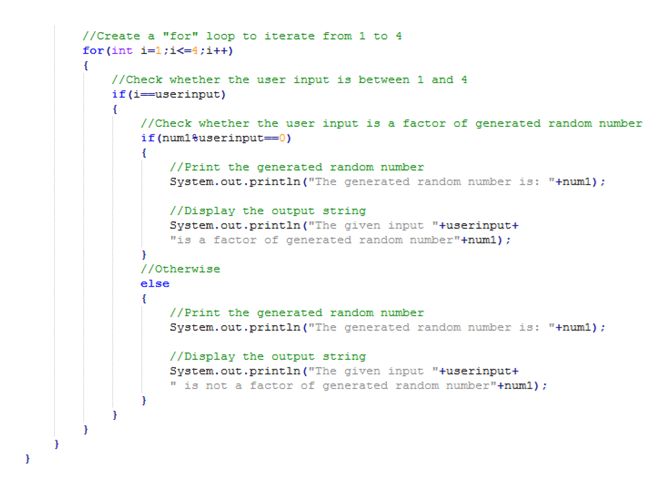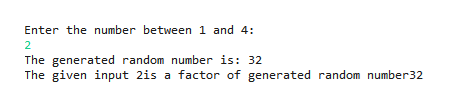# Hello, I am using the Intro to Java programmming and data structures by Y. Daniel Liang.Our professor wants us to generate a random number in java between 1 and 100. Then prompt the user for a number between 1 and 4. Next, the program will determine if the number entered by the user is a factor of the random number. I feel like I have some parts of the code down pack but not all of it. Thus far I have: import java.util.Scanner;public class Module_Project3 {public static void main(String[] args) {Scanner input = new Scanner(System.in);int num1 = (int)(Math.random()*100+1);int num2 = (int)(Math.random()*100+1);int num3 = (int)(Math.random()*100+1);int num4 = (int)(Math.random()*100+1);

Question
15 views

Hello, I am using the Intro to Java programmming and data structures by Y. Daniel Liang.

Our professor wants us to generate a random number in java between 1 and 100. Then prompt the user for a number between 1 and 4. Next, the program will determine if the number entered by the user is a factor of the random number.

I feel like I have some parts of the code down pack but not all of it. Thus far I have:

import java.util.Scanner;
public class Module_Project3
{

public static void main(String[] args)
{
Scanner input = new Scanner(System.in);

int num1 = (int)(Math.random()*100+1);
int num2 = (int)(Math.random()*100+1);
int num3 = (int)(Math.random()*100+1);
int num4 = (int)(Math.random()*100+1);

check_circle

Step 1

A java program to check the user’s input is a factor of random number generated is given below;

Program:

//Import a required package

import java.util.Scanner;

//Create a class

public class RandFactor

{

//Define a main() function

public static void main(String[] args)

{

/*Create an object for Scanner class to get the input from the user*/

Scanner input = new Scanner(System.in);

/*Generate a random number between 1 and 100 using random() method*/

int num1 = (int)(Math.random()*100+1);

//Print the string

System.out.println("Enter the number between 1 and 4: ");

//Get the input from the user using next() method

int userinput=input.nextInt();

//Create a "for" loop to iterate from 1 to 4

for(int i=1;i<=4;i++)

{

//Check whether the user input is between 1 and 4

if(i==userinput)

{

/*Check whether the user input is a factor of generated random number*/

if(num1%userinput==0)

{

//Print the generated random number

System.out.println("The generated random number is: "+num1);

//Display the output string

System.out.println("The given input "+userinput+"is a factor of generated random number"+num1);

}

//Otherwise

else

{

//Print the generated random number

System.out.println("The generated random number is: "+num1);

//Display the output string

System.out.println("The given input "+userinput+" is not a factor of generated random number"+num1);

}

}

}

}

}

Screenshot of program #1:

Step 2

Screenshot of program #2:help_outlineImage Transcriptionclose/Create a "for" loop to iterate from 1 to 4 i=1; 1< - 4; 1 ++ for (int //Check whether the user input is between 1 and 4 if (i-userinput) 1/Check whether the user input is a factor of generated random number if (numleuserinput=0) //Print the generated random number System.out.println ("The generated random number is: "+numl) /Display the output string System.out.println ("The given input "+userinput+ "is a factor of generated random number"+numl) //otherwise else //Print the generated random number System.out.print ln ("The generated random number is: "+num1); //Display the output string System.out.println ("The given input "+userinput "is not a factor of generated random number"+numl); fullscreen
Step 3

Screenshot of output #1...help_outlineImage TranscriptioncloseEnter the number between 1 and 4: 2 The generated random number is: 32 The given input 2is a factor of generated random number32 fullscreen

### Want to see the full answer?

See Solution

#### Want to see this answer and more?

Solutions are written by subject experts who are available 24/7. Questions are typically answered within 1 hour.*

See Solution
*Response times may vary by subject and question.
Tagged in

### Java